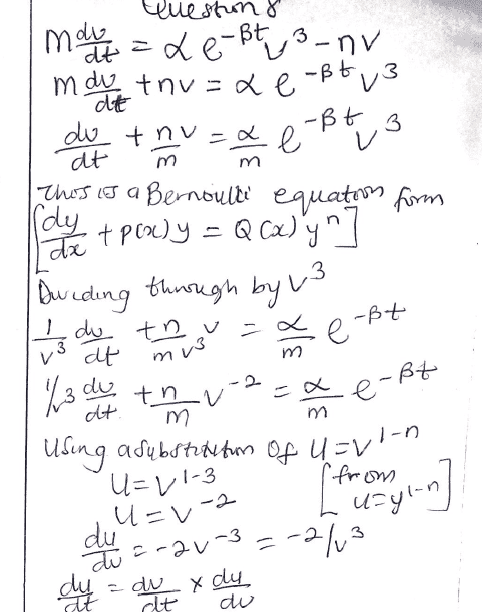# ODE Methods for Physicists (related question)

• profgabs05
profgabs05
Homework Statement
A mass 𝑚 is accelerated by a time-varying force 𝛼 𝑒𝑥𝑝(−𝛽𝑡)𝑣3, where v is its velocity. It also experiences a resistive force 𝜂𝑣, where 𝜂 is a constant, owing to its motion through the air. The equation of motion of the mass is therefore
𝑚𝑑𝑣/𝑑𝑡= 𝛼 𝑒𝑥𝑝(−𝛽𝑡)𝑣^3 − 𝜂𝑣.
Find an expression for the velocity v of the mass as a function of time, given that it has an initial velocity 𝑣0
Relevant Equations
𝑚𝑑𝑣/𝑑𝑡= 𝛼 𝑒𝑥𝑝(−𝛽𝑡)𝑣^3 − 𝜂𝑣.#### Attachments

Mentor
$$\frac{1}{v^3}\frac{dv}{dt}=-\frac{1}{2}\frac{dv^{-2}}{dt}$$

profgabs05
$$\frac{1}{v^3}\frac{dv}{dt}=-\frac{1}{2}\frac{dv^{-2}}{dt}$$
$$\frac{dx^n}{dx}=nx^{n-1}$$